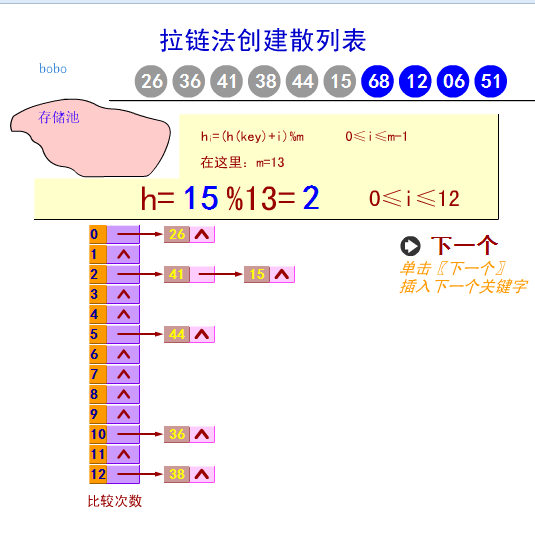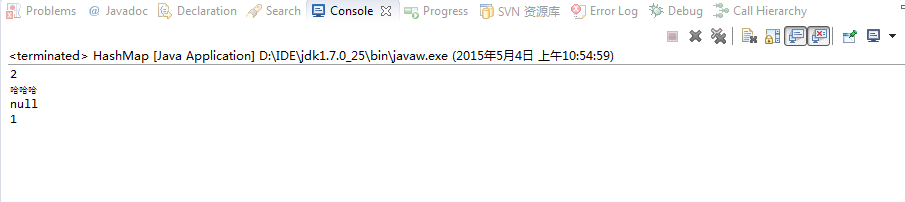# 大数据处理算法三：分而治之/hash映射 + hash统计 + 堆/快速/归并排序

IP 32位的，最多有个2^32IP。同样可以采用映射的方法，比如模1000，把整个大文件映射为1000个小文件，再找出每个小文中出现频率最大的 IP（可以采用hash_map进行频率统计，然后再找出频率最大的几个）及相应的频率。然后再在这1000个最大的IP中，找出那个频率最大的IP，即 为所求。

第二步借用堆排序找出最热门的10个查询串：时间复杂度为N'*logK。维护一个K(该题目中是10)大小的小根堆，然后遍历3百万个Query，分别和根元素进行对比（对比value的值），找出10value值最大的query
最终的时间复杂度是：ON） + N'*OlogK），（N1000万，N’为300万）

1. HashMap的数据结构

数组

哈希表有多种不同的实现方法，我接下来解释的是最常用的一种方法—— 拉链法，我们可以理解为“链表的数组”index=hashCode(key)=key％16

/**
* 自定义 HashMap
* @author JYC506
*
* @param <K>
* @param <V>
*/
public class HashMap<K, V> {

private static final int CAPACTITY = 16;

transient Entry<K, V>[] table = null;

@SuppressWarnings("unchecked")
public HashMap() {
super();
table = new Entry[CAPACTITY];
}

/* 哈希算法 */
private final int toHashCode(Object obj) {
int h = 0;
if (obj instanceof String) {
return StringHash.toHashCode((String) obj);
}
h ^= obj.hashCode();
h ^= (h >>> 20) ^ (h >>> 12);
return h ^ (h >>> 7) ^ (h >>> 4);
}
/*放入hashMap*/
public void put(K key, V value) {
int hashCode = this.toHashCode(key);
int index = hashCode % CAPACTITY;
if (table[index] == null) {
table[index] = new Entry<K, V>(key, value, hashCode);
} else {
for (Entry<K, V> entry = table[index]; entry != null; entry = entry.nextEntry) {
if (entry.hashCode == hashCode && (entry.key == key || key.equals(entry.key))) {
entry.value = value;
return;
}
}
Entry<K, V> entry2 = table[index];
Entry<K, V> entry3 = new Entry<K, V>(key, value, hashCode);
entry3.nextEntry = entry2;
table[index] = entry3;

}
}
/*获取值*/
public V get(K key) {
int hashCode = this.toHashCode(key);
int index = hashCode % CAPACTITY;
if (table[index] == null) {
return null;
} else {
for (Entry<K, V> entry = table[index]; entry != null; entry = entry.nextEntry) {
if (entry.hashCode == hashCode && (entry.key == key || key.equals(entry.key))) {
return entry.value;
}
}
return null;

}
}
/*删除*/
public void remove(K key){
int hashCode = this.toHashCode(key);
int index = hashCode % CAPACTITY;
if (table[index] == null) {
return ;
} else {
Entry<K, V> parent=null;
int i=0;
for (Entry<K, V> entry = table[index]; entry != null; entry = entry.nextEntry) {
if (entry.hashCode == hashCode && (entry.key == key || key.equals(entry.key))) {
if(i==0){
table[index]=null;
}
if(parent!=null){
parent.nextEntry=entry.nextEntry;
}
entry=null;
return ;
}
i++;
parent=entry;
}
}
}
public static void main(String[] args) {
HashMap<String,String> map=new HashMap<String,String>();
for(int i=0;i<10000;i++){
map.put(Integer.toString(i), Integer.toString(i));
}
map.put("1", "2");
map.put("3", "哈哈哈");
System.out.println(map.get("1"));
System.out.println(map.get("3"));
map.remove("1");
System.out.println(map.get("1"));
for(int i=0;i<10000;i++){
String s=    map.get(Integer.toString(i));
if(s==null){
System.out.println(i);
}
}
}

}

class Entry<K, V> {
K key;
V value;
int hashCode;
Entry<K, V> nextEntry;

public Entry(K key, V value, int hashCode) {
super();
this.key = key;
this.value = value;
this.hashCode = hashCode;
}

}

/* 字符串hash算法 */
class StringHash {
public static final int toHashCode(String str) {
/* 我用java自带的 */
return str.hashCode();
}
}